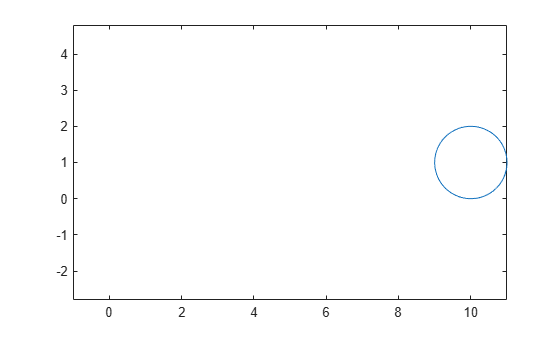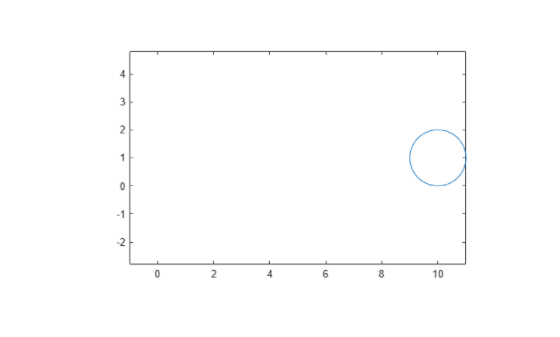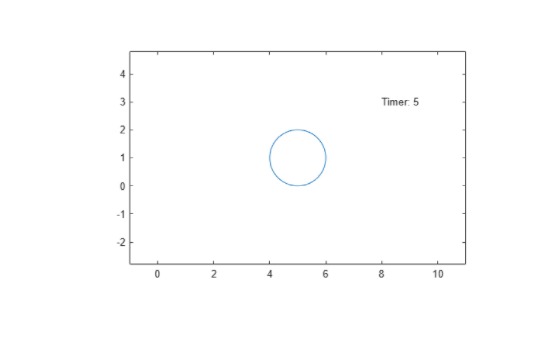# animationToFrame

Return structure of frames from animation objects

Since R2019a

## Syntax

``frames = animationToFrame``
``frames = animationToFrame(fig)``
``frames = animationToFrame(___,Name,Value)``

## Description

example

````frames = animationToFrame` returns a structure array of frames from animation objects. The animation objects must be created using the `fanimator` function.```

example

````frames = animationToFrame(fig)` returns a structure array of frames from animation objects in the figure `fig`.```

example

````frames = animationToFrame(___,Name,Value)` uses the specified `Name,Value` pair arguments. Use this option with any of the input argument combinations in the previous syntaxes.```

## Examples

collapse all

Create an animation of a moving circle, and return specific frames of the animation.

First, create two symbolic variables, `t` and `x`. The variable `t` defines the time parameter of the animation. Use `t` to set the center of the circle at `(t,1)` and `x` to parameterize the perimeter of the circle within the range `[-pi pi]`. Create the circle animation object using `fanimator`. Set the x-axis and y-axis to be equal length.

```syms t x fanimator(@fplot,cos(x)+t,sin(x)+1,[-pi pi]) axis equal```

By default, `fanimator` generates an animation object with 10 frames per unit time within the range of `t` from 0 to 10. The default animation object contains a total of 101 frames. Use the command `playAnimation` to play the animation.

Next, return a structure array of frames from the animation object by using `animationToFrame`.

`frames = animationToFrame````frames=1×101 struct array with fields: cdata colormap ```

The structure `frames` contains two fields. The `cdata` field stores the image data as an array of `uint8` values.

Reconstruct the animation frames by using the `imshow` function. For example, display the 50th frame and the last frame of the animation.

`imshow(frames(50).cdata)``imshow(frames(101).cdata)`Create a moving circle animation object and a timer animation object. Return the generated animation frames in reverse order.

First, create two symbolic variables, `t` and `x`. The variable `t` defines the time parameter of the animation. Create a figure window for the animation.

```syms t x fig1 = figure;```

Create a circle animation object using `fanimator`. Use `t` to set the center of the circle at `(t,1)` and `x` to parameterize the perimeter of the circle within the range `[-pi pi]`. Set the x-axis and y-axis to be equal length.

```fanimator(@fplot,cos(x)+t,sin(x)+1,[-pi pi]) axis equal```

Next, use the `text` function to add a piece of text to count the elapsed time. Use `num2str` to convert the time parameter to a string.

```hold on fanimator(@(t) text(8,3,"Timer: "+num2str(t,2))) hold off```

By default, `fanimator` creates stop-motion frames with 10 frames per unit time within the range of `t` from 0 to 10. The default animation object contains a total of 101 frames. Use the command `playAnimation` to play the animation.

Next, return a structure array of frames from the animation in figure `fig` by using `animationToFrame`. Return the animation frames in reverse order by setting the `'Backwards'` option to `true`. Set the frame rate per unit time to 2 to return a total of 21 frames.

`frames = animationToFrame(fig1,'Backwards',true,'FrameRate',2)````frames=1×21 struct array with fields: cdata colormap ```

The structure `frames` contains two fields. The `cdata` field stores the image data as an array of `uint8` values.

Reconstruct the animation frames by using the `imshow` function. For example, display the first frame and the 11th frame of the animation in a new figure window.

```fig2 = figure; imshow(frames(1).cdata)````imshow(frames(11).cdata)`## Input Arguments

collapse all

Target figure, specified as a `Figure` object. For more information about `Figure` objects, see `figure`.

### Name-Value Arguments

Specify optional pairs of arguments as `Name1=Value1,...,NameN=ValueN`, where `Name` is the argument name and `Value` is the corresponding value. Name-value arguments must appear after other arguments, but the order of the pairs does not matter.

Before R2021a, use commas to separate each name and value, and enclose `Name` in quotes.

Example: `'Backwards',true,'AnimationRange',[-2 5]`

Range of the animation time parameter, specified as a two-element row vector. The two elements must be real values that are increasing.

Example: `[-2 4.5]`

Frame rate, specified as a positive value. The frame rate defines the number of frames per unit time when you returning animation frames as a structure array.

Example: `20`

Backward option, specified as a logical value (boolean). If you specify `true`, then the function returns the animation frames backwards or in reverse order.

Example: `true`

## Output Arguments

collapse all

Animation frames, returned as a structure array with two fields:

• `cdata` — The image data stored as an array of `uint8` values. The size of the image data array depends on your screen resolution.

• `colormap` — The colormap. On true color systems, this field is empty.

The `animationToFrame` function returns a structure of animation frames in the same format as the output returned by the `getframe` function.

## Version History

Introduced in R2019a